## Wednesday, December 9, 2015

### Guide to the EU – Chapter 6 Currents, Filaments and Pinches

We now get into the meat of the empirical  aspects of plasmas.  Our theoretical tools are wonderful but provide models that fall apart easily with a slight disturbance.  Yet we do find repetitive processes at work and causes that can be tracked as well.  All good but straining on our computer power to model.

In the meantime  empirical knowledge is intuitive and surprisingly stable in its own right.  Thus we have advanced steadily.

There just too many active centers in a plasma to allow predictive methods to ever be either exact or sufficiently inexact as well. It is a little like building large stone structures where you over engineer it all.
.

Essential Guide to the EU – Chapter 6 Currents, Filaments and Pinches

Planetary nebulas often exhibit characteristic bi-polar symmetries with a centered plasma pinch, polar jets, and an equatorial torus. Image courtesy NASA, ESA and the Hubble Heritage Team

https://www.thunderbolts.info/wp/2011/12/06/essential-guide-to-the-eu-chapter-6/

6.1  Thermal Motion and Current

It is important to distinguish between random thermal motion and uniform linear motion in a plasma. The latter is an electric current which flows due to the presence of an electric field.

The random thermal motion is measured by the temperature of the plasma, or by the temperatures of the ions and electrons separately if their temperatures are different. This motion, being a motion of charged particles, is also a form of current, but one which oscillates about an average position, as opposed to moving in one direction only. Strictly speaking, temperature can only be an accurate measure of the energy if the distribution of velocities of individual particles is Maxwellian, that is, if the distribution is equivalent to that which would result from elastic collisions between the particles.
Uniform linear motion results from an electric field and represents a drift current. All particles with the same sign charge (positive or negative) move in the same direction under the influence of the electric field. That is, in a plasma, where there are roughly equal numbers of positive and negative particles (“net neutrality”), we find the positively charged particles moving together in a direction opposite that of the electrons’ motion.

The particles all have kinetic energy, which may be high, but they do not have a temperature as a result of this linear motion. That’s because temperature is only used to measure the energy of particles with random velocities, undergoing collisions. As both types tend to move along more or less aligned or parallel trajectories, and plasma densities are relatively low, collisions are less common and Maxwellian collision conditions are not obtained.

Both types of motion exist simultaneously wherever a current is flowing. The current motion or drift of the particles is superimposed on the random motions. Another way of looking at this is to think of the mean position of the random motion as moving with the drift velocity in the direction of the current.

6.2  Electron and Ion Currents

We have seen that the electrons acquire much higher velocities than ions due to their smaller mass.

However, an electron carries the same magnitude of (negative) charge as a positively charged proton, the lightest form of ion. Therefore, the higher velocity of the electrons means that they are more effective than the ions at carrying current in a plasma.

The ratio of the electron current to the ion current in a non-relativistic plasma current is proportional to the square root of the inverse ratio of the masses. For the lightest positive ion, a proton, this means that the electron current is around 43 times greater than the ion current.  [ If the mass of an electron is taken as 1, the mass of a proton would be about 1836 times greater):  √(1836 ÷ 1) = 42.85 ]  In many situations, it is the motion of the electrons which determines the plasma behavior.

6.3  Current in Laboratory Discharge Tubes

Plasma has been studied in laboratory experiments for over a hundred years, and a vast amount of experimental data and analysis is now available. One of the fundamental experiments involves a Glow Discharge Tube in which a current is passed through a low-pressure gas such as mercury vapor. This causes ionization of the gas and creation of a plasma within the tube.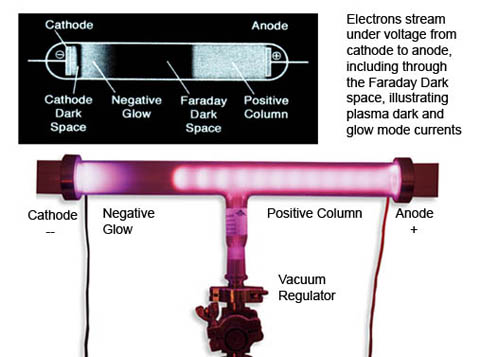Evacuated (low pressure gas) tube with anode and cathode and high-voltage power source. Image credit: Wiki Creative Commons
6.4  Glow Discharge Tubes

Many descriptions of discharge tubes are available and will not be repeated here in detail. The salient points for the present purposes are as follows:
1. Within the tube, there are visible bands along the axis wherein the plasma is seen to glow, interspersed with ‘dark’ bands where there is no such glow. The different bands represent two of the three possible modes of operation of plasma when carrying a current.
1. The dark bands represent, unsurprisingly, the Dark Current Mode. In these regions the electron velocity is below that necessary to cause visible excitation of the atoms of neutral gas, although ionization will start to occur at higher currents. However, radiation will be given off at wavelengths outside the visible even in the Dark Current Mode and so may be detected by non-optical means.
1. The glowing bands represent the Normal Glow Mode. Here, the velocity of the electrons causes ionization to occur. The glow is caused by radiation from the electrons of neutral atoms after they have been excited by collisions with fast free electrons.
1. The third possible mode of plasma operation is the Arc Mode, familiar in painfully bright welding applications or lightning, for example.
1. Returning to the glow discharge tube, one might expect that the potential difference between the electrodes would cause a uniform electric field along the length of the tube. However, the plasma behaves differently.
1. It is found that a Double Layer (DL) develops in the tube which modifies the externally applied electric field between the anode and cathode. The DL forms in such a way that the majority of the potential drop occurs across the DL. Away from the DL region, much of the remainder of the plasma is a glow discharge region known as the positive column. This can extend for a significant part of the length of the discharge tube.
1. Within the positive column there are approximately equal numbers of electrons and ions. The plasma here is therefore quasi-neutral. Because most of the potential drop occurs across the DL, only a small but constant voltage gradient, or electric field, exists within the positive column.
1. There appear to be analogies between the positive column in a discharge tube and the plasma within the Sun’s heliosphere.
1. Another result of the discharge tube experiments is also relevant to our discussion of plasma behavior and will be discussed in the next section.
6.5  The Current Density–Voltage Curve

If the current density J (x-axis) is plotted against the voltage V (y-axis) in a discharge tube (current density is the current divided by the area of the discharge tube), then it is found that the three different plasma glow modes correspond to three different sections of a discontinuous graph, known as the current vs voltage or J-V curve.

In the dark discharge mode, the J-V curve rises with increasing voltage, although not regularly. Once the voltage reaches a high enough value, ionization begins and the current starts to rise very rapidly for very little increase in voltage.

The discharge will then change rapidly into the glow discharge mode. This is accompanied by a dramatic step change in the voltage. The voltage drops because, when large numbers of electrons have been produced by ionization, only a small voltage is needed in order to generate a large current.

A very significant effect often occurs in the lower current density part of the glow discharge region. The voltage actually decreases with increasing current density. In other words, the plasma finds it more efficient to transmit the current at a higher current density because the voltage drop is less.
At still higher current densities, the voltage increases again, meaning that the glow discharge section of the J-V curve has a minimum at a particular value of current density. This minimum represents the point of lowest resistance for transmission of the total current. In cosmic plasmas, this effect may be significant in causing the formation of current filaments by confining the current within a particular cross-sectional area.

Similarly, in the extremely bright arc discharge mode, the voltage once again decreases with increasing current density. If plasma is forced into the arc mode, it will again tend to filament in order to reduce the voltage drop.

6.6  Current Filamentation

Filamentation is observed to be a normal behavior mode for currents in a plasma, as evidenced by the J-V curve and by physical structures in space itself. A paper by Dr. Anthony Peratt regarding filamentation can be found here.

In particular, current sheets (which we will consider later) tend to break up into individual filaments due to the development of vortices. These vortices are somewhat similar to those found in fluid flows with adjacent layers of different flow velocities (Kelvin-Helmholtz instabilities).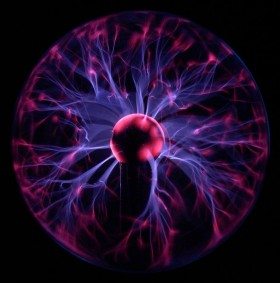A small plasma lamp’s central cathode is surrounded by an excited spherical current sheet which is attracted to the outer glass shell (anode). Where the current extends outward from the surface sheet it forms filamentary structures as it propagates through the low pressure medium within the lamp. Photo credit: Luc Viatour /
www.lucnix.be

Clearly, the conditions inside a current filament are going to be different to those in the rest of the plasma. This causes a current-free double layer (CFDL) to form at the boundary of the filament in the normal way, such that the faster electrons are confined to the filament by the electric field within the DL.

We can now see that filaments are current-carrying elongated plasma cells with CFDLs at their boundaries.

Evidence of filaments_and_electric_currents in space is widespread. Filamentary structure is acknowledged by most astronomers to exist at all levels, from the solar system to galactic and intergalactic scales. The only area of disagreement between the Electric Model and the Gravity Model is whether these filaments are current-carrying structures, naturally following the laws of plasma electrodynamics, or somehow fluid ‘jets’ thousands of light-years long, gravitationally driven in accordance with computer simulations of the hypothesized gravity forces due to cold dark matter (CDM).

In a fluid, jets tend to dissipate rapidly into low-velocity plumes.An aircraft’s turbines expel jets of gas, seen here as contrails of ice crystals precipitating some distance aft of the engines, which quickly expand and decelerate to a stop in the upper atmosphere

However, some jets in space, for example the 4,000 light-years-long jet from the elliptical galaxy M87, appear to remain in the jet state for enormous distances before dissipating into a plume. This might indicate that the jets are not fluid jets but electrical filaments.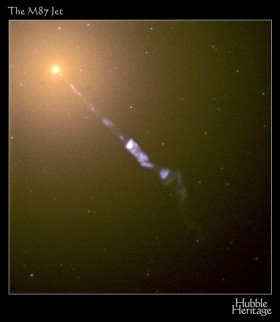The jet from galaxy M87. Galaxy is the bright knot, upper left, in visible light (reddish); the jet extends down and to the right, seen here in UV light (white and blue). Image credit: NASA/ Hubble

A significant paper titled, “Measurement of the Current in a Kpc-Scaled Jet” was published in 2011 in arXiv by Kronberg, Lovelace, et al, based on their investigations of a jet emanating from radio galaxy 3C303

If we assume they are electric filaments, then we need to know what theory and experiment might tell us about how electric filaments keep their shape over astronomical distances. This is discussed next.

6.7  Current Pinches

Any current I flowing in a conductor or filament will cause a magnetic field B around it. The lines of equal magnetic force will be in the form of rings around the axis of the current. The magnetic force will decrease with radial distance from the axis.

From consideration of the Lorentz Force, it can be shown that the interaction of the current I with its own magnetic field B will cause a pressure radially inward on the current filament, written as I × B (that is, “I cross B” in vector terminology). This is called a ‘pinch’ or ‘z-pinch’ (when defining the current flow as parallel with the ‘z’ co-ordinate’s direction).

In a metal conductor, the I × B pressure is resisted by the atomic ion lattice. In a plasma current, the pressure can be balanced by the pressure of the plasma inside the filament. This results in a steady state where the current can flow axially across its own azimuthal or circling magnetic field. The balancing equation is known as the Bennett Pinch equation.

Lab demonstrations can use the pinch effect to crush aluminum cans by applying a strong magnetic field very quickly. The can is crushed before the pressure in the can is able to build up sufficiently to resist the pinch force. Magnetic field forces in lightning can create an inward pinch that will crush a solid copper grounding rod.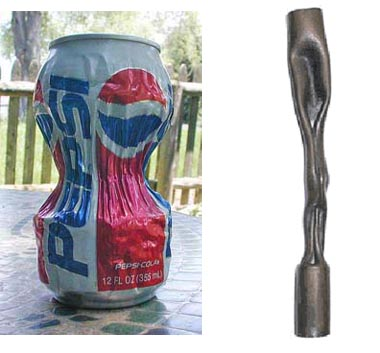Left: The field generated by a fast 2 kj discharge through 3-turn heavy wire crushed this can. Right: Nature’s lightning z-pinch deformed this metal rod. Images credit: Wiki Creative Commons

6.8  Field-Aligned Currents

In space, the neutral gas pressure is usually negligible, and so the balance between the I × B force and the pressure force cannot occur. The only way the situation can be resolved is for the I × B force to disappear. This implies that I and B (current direction and magnetic field direction) are parallel and, by vector algebra, the cross product is zero.

If other magnetic fields are present, as they are known to be through much of cosmic space, then the I × B force must be calculated using the total magnetic field, that is, by adding the current’s own B to the general B, added using vector algebra.

Thus in a space plasma, the current I and the total magnetic field B realign so as to be parallel. In other words, the current follows the magnetic field: it is a ‘field-aligned’ current.

Even if there is no external magnetic field, any small elements of current flowing in a plasma will tend to accumulate naturally into larger currents which generate their own magnetic fields and so preserve the filament of current.

What happens is that electrons nearer the centre of the filament flow in almost straight lines and generate an azimuthal magnetic field around them. Electrons further from the centre are influenced by this azimuthal component of the magnetic field and move in a more helical path aligned with the main current direction. This helical motion creates the straighter magnetic field lines near the axis, as shown in the following diagram. The nearer the centre of the filament, the straighter are the magnetic field lines and the paths of the electrons.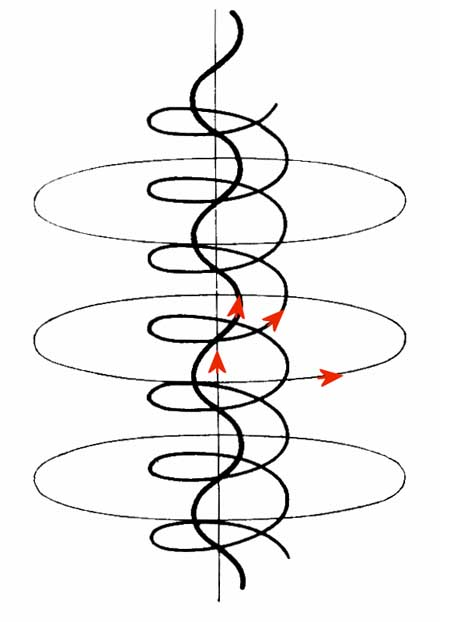Electron flows in a field-aligned current at varying distances from the center of the current filament. Image credit: Wiki Commons

Any individual electron in the current is thus flowing along the magnetic field direction in its own vicinity, but collectively the filament is preserved even without an external magnetic field. This means that very large currents can be assembled out of small current elements and transmitted over huge distances.

Another way of looking at this is to consider the electrical resistance of the plasma. Current flowing across the magnetic field direction will experience more resistance than current flowing along the magnetic field direction because of the U × B term in the Lorentz Force Law. Effectively, the parallel resistance is less than the perpendicular resistance, so the current tends to flow in alignment with the magnetic field.

6.9  Self-Constriction of Currents

Detailed mathematical analysis shows that I and B interact in such a way that both I and B tend to spiral parallel to each other around an axis aligned with the external B. The net effect is that I and B both follow a helical path aligned with the direction of the external B field.

It is also found that the interaction of the axial and azimuthal (ring) components of the helical I and B cause both I and B to be largely confined to a cylinder of definite radius centered on the axis.

To summarize, the absence of significant pressure in space plasmas causes currents to flow in cylindrical filaments aligned with the general magnetic field direction. Within the cylindrical filament, both the current and the magnetic field will spiral around the axis of the cylinder whilst remaining parallel to each other.

Note that if for any reason the parallel alignment between I and the total B is disturbed, then an I × B force will arise and cause either radial compression or radial expansion, depending on which of the two components is more axial. Thus pinching of a filament could occur because, for example, of changes in the fields through which the current filament was flowing.

6.10  Stability of Current Filaments

Another significant factor emerges from the mathematical analysis. The force-free or field-aligned arrangement is a minimum energy state for the current to flow in. This means that the field-aligned arrangement is inherently stable. Unless disturbed by external factors, currents will tend to remain aligned with the magnetic field.

We can now see how field-aligned currents can persist over vast distances. Field-aligned currents are therefore a much more likely explanation of the collimated (parallel-flow) ‘jets’ seen to be extending for hundreds to thousands of light-years than is the Gravity Model explanation based on conventional fluid flows (refer to 6.6 above).  The UK radio observatory at Jodrell Bank has collected a wide variety of images of galactic jets seen in radio wavelengths, in their Atlas of DRAGNs (Double Radiosource Associated with Galactic Nuclei)  One such image is depicted below, showing a typical double jet:

The confinement of field-aligned filamentary currents to definite cylinders of current by electromagnetic forces is also consistent with the falling characteristic of the J-V curve seen in laboratory experiments in discharge tubes. If the plasma is in Glow Mode, which in space plasmas may mean a glow in wavelengths outside the visible range, then the radius of the current cylinder will be determined by a combination of the effects of the electric and magnetic fields and the shape of the current density-Voltage curve. Read more about the filamentation process in dense cosmic z-pinches in this_paper by Russian physicists A.B Kukushkin and V.A. Rantsev-Kartinov of the Kurchatov Institute, Moscow.

6.11  Condensation of Matter

A further effect related to the I × B force can also be determined by analysis. Suppose that the current I is caused by an electric field E. Now consider the force arising from the interaction of E and B. Remember that I tends to become aligned with the total B due to the forces on the current itself. Then the E causing the current will not be entirely aligned with the total B, which is the vector sum of the external magnetic field through which the current flows and the azimuthal magnetic field generated by the current itself.

As with the I × B force, there is also an E × B force, whenever E is not parallel to B. This E × B force acts on charged particles in the current cylinder and causes both ions and electrons to move towards the centre of a filament. Plasmas often contain a high proportion of charged dust grains, which will also be drawn into the filament. Viscous drag between the charged particles and neutral atoms will tend to draw the neutral atoms towards the filament as well.

Therefore, current filaments in space will tend accumulate matter in them as a result of the misalignment of the electric field causing the current and the total magnetic field.

Remembering that pinches can occur if any misalignment of I and B occurs, any matter that has been drawn into the filament will also be compressed if a misalignment of I and B occurs. If the pinch force is large enough, it can fragment the filament into discrete spherical or toroidal plasmoids along the axis of the current. Any matter in the pinch zone would then become compressed into the same form.

Because the electromechanical forces are vastly stronger than gravity, this mechanism offers a means by which diffuse matter can be accumulated and compressed in a much more efficient way than gravitational compression of diffuse clouds of fine dust particles.

[ this provides a protocol for matter accumulation that satisfies the needs of star making - arclein ]

Of course, once the matter has been sufficiently compressed and if it is neutralized by recombination of ions and electrons, then the electromagnetic forces may be reduced to the point that gravity becomes significant and continues the compression started by the electromagnetic forces.

6.12  Marklund Convection

In the case of a cylindrical current, the E × B force is radially inwards and results in the self-constriction of a current filament, as we have seen. This results in an increase in the particle density near the axis of the current. Two things can then happen.
• The first is that radiative cooling from the regions of increased density can result in a temperature decrease nearer the center, contrary to the increase one might intuitively expect from increasing the density.
• The second is that recombination of ions and electrons starts to occur.
Every chemical element has a particular energy level, known as its ionization energy, at which it will either ionize or recombine. This is analogous to the boiling point of a liquid such as water: at a particular temperature, the phase or state of the matter will change from one state to another.

If the kinetic energy of motion is equated with the ionization energy, then a characteristic velocity, known as the Critical Ionization Velocity (CIV), can be derived for each element. Because temperature is a measure of thermal energy, CIV can be related to temperature. The CIV values of elements commonly found in space are not distributed randomly but are grouped into four distinct bands around certain velocity values. Within each band, all the elements in that band have similar CIVs to each other.

In the vicinity of a field-aligned current, the E × B force causes a radial drift of ions and electrons towards the cooler central axis. Because of their differing CIVs, different ions will recombine at different radii as they move towards the centre and enter progressively cooler regions.

This process is known as Marklund Convection after the Swedish physicist who discovered it, Göran Marklund.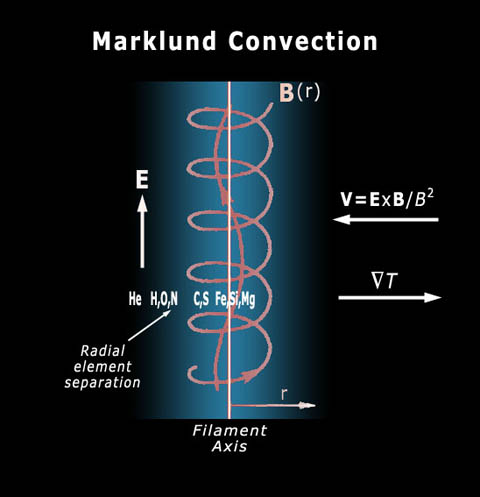Marklund convection and sorting in a magnetically pinched current. Image courtesy of Wal Thornhill, www.holoscience.com

The net result is that Marklund Convection sorts any elements present in the locality into different groups according to their ionization potentials. The groups of elements are arranged in cylindrical shells at different radii within a cylindrical field-aligned current.

As hydrogen has a high CIV compared to the other elements, it will recombine first, in a cylindrical shell of larger radius than the shells of the other elements.

This type of electrical sorting may be responsible for some of the non-random distribution of elements that we observe in the cosmos. In particular, it may explain the preponderance of neutral hydrogen in thread-like structures throughout the galaxy that have been detected by radio telescopes.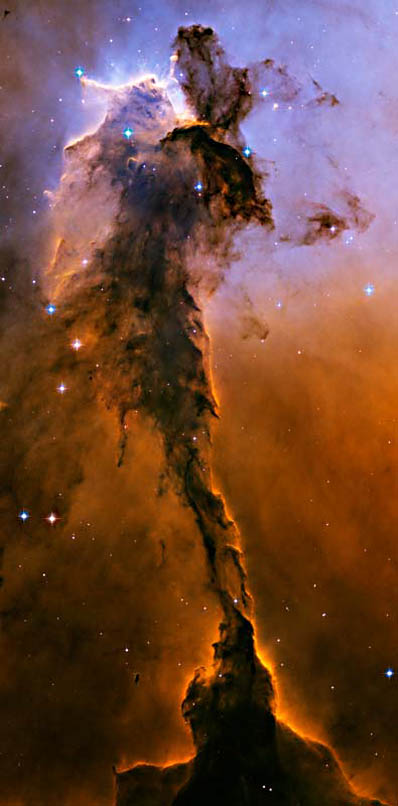Could this Eagle Nebula image by the Hubble Space Telescope be an illustration of a cosmic magnetic pinch and resultant dusty plasma surrounded by a hydrogen-helium environment?

End of Chapter 6